# Using CVX to Optimize SOCP Problem

I’m trying to optimize the SOCP problem in the
paper using CVX with SeDuMi however at the end of the optimization the variables are all zero(0). It is noted in the command window “There is no sensible solution”.

and here is my matlab code

``````n = 200;
cvx_begin

variable r nonnegative
variable e(n) nonnegative
variable w(n) nonnegative

minimize( r + C*sum(e) )

subject to

r > 0
e >= 0

for i = 1 : 200
norm( M*K(:,i) - M*K*w ) <= r + e(i)
end

cvx_end
``````

anyboyd help me??

You haven’t provided a reproducible problem, complete with data input.

Can you at least show the complete solver and CVX output? Have you tried SDPT3? Note that `r > 0` will be treated by CVX as being `r >= 0`, and actually is redundant (although I don’t think that causes any harm), as also is `e >= 0`, given your declaration of them as nonnegative. If you need r to be strictly positive, you can use `r >= small_number`, where `small_number` is big enough to ensure r is strictly positive, given solver tolerance.

1 Like
• Yes, I need r to be strictly positive and e need to be nonnegative , I fixed this in my code as you mentioned above.

• In my code variable M is
M = sqrt(n)*inv(big_omega)*A
n–> 200(constant), big_omega --> [nXn] matrix, A --> [nXn] matrix. (as in the optimization problem)

All the variables are given in the replies below in Matlab form.

• There is one case that i dont understand. I normally have 201 linear inequality constraints. However, when i run the code step by step, the number of the inequality constraints and equality constraints increase in each iteration in the for loop(where the SOCP constraints are). I don’t know why?

Here is my code(modified):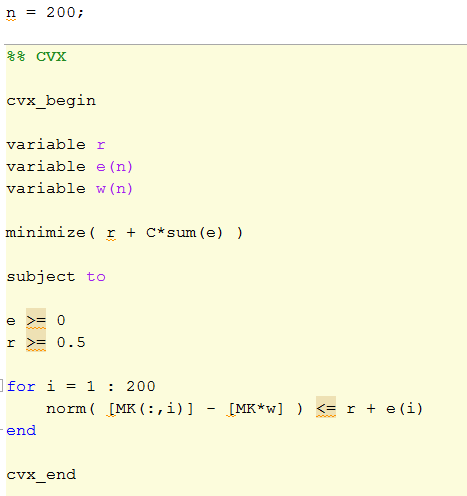Here is the CVX and Solver(SeDuMi) output(command window):

Here is the all variables when i’m using SeDuMi: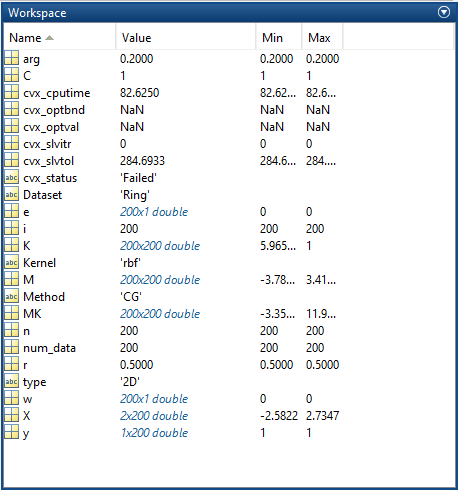Here is the optimization variables when i’m using SeDuMi:

Here is the CVX and Solver(SDPT3) output(command window):

Here is the all variables when i’m using SDPT3: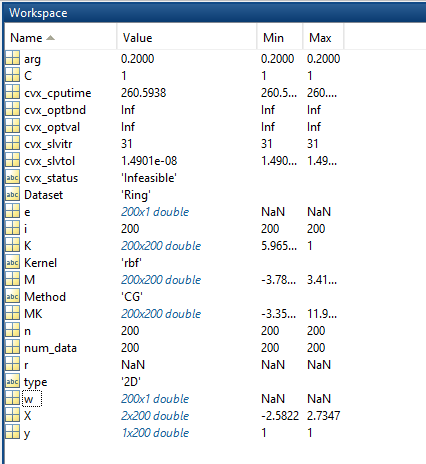Here is the optimization variables when i’m using SDPT3:

Sedumi had numerical difficulty, so the “solution”, i.e., the values of CVX variables after execution, is not meaningful. SDPT3 basically declared the problem to be infeasible. I suspect that the values of M and K result in a numerically ill-behaved problem - this could potentially result in a false conclusion of infeasibility, as happened using SDPT3, even though a human observer can clearly see the problem is feasible. Perhaps your problem is inherently extremely ill-conditioned - maybe Omega is nearly singular? You ought to look at the span of magnitudes of elements of M and K, which could be a contributor, apart from ill-conditioning, to numerical difficulties.

It may be that there is s a more numerically stable reformulation, or if not, that you might need a quad precision or higher solver (not available under CVX). If you have the capability to use MOSEK or GUROBI under CVX, you could try them, as I think those solvers are more numerically robust than sedumi or SDPT3, and therefore can handle somewhat nastier problems before running into numerical difficulties they can’t handle.

1 Like

If you look at the SeDuMi output then it gives up after 1 iteration. That typically mean you did something wrong when formulating the problem unless it run out of space.

SeDuMi assumes the objective is c’ x. It seems like ||c|| is HUGE. I.e. your problem has HUGE scaling issues.

1 Like

There is one case that i dont understand. I run the code step by step and analyze the constraints in the one of th evariable of cvx. In theorr, I have 201 linear inequality constraints. However, when i run the code step by step, the number of the inequality constraints and equality constraints increase in each iteration in the for loop(where the SOCP constraints are). CVX takes the SOCP constraint as if it is eauality and inequality constraints. I have more than 40000 equality and inequality constraints at the line"cvx_end". I don’t know why? Is it ok?

How are you running the code “step by step”? I don;t know what that means in this case.

1 Like

I put a break point at the line of SOCP constraint.

and in each iteration of the for loop i get

for i = 1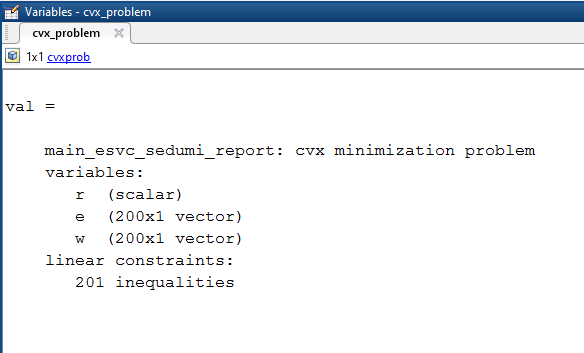for i = 2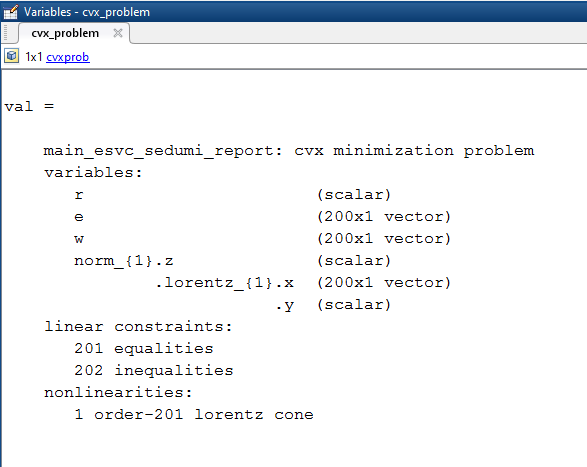and the last
for i = 200

i have

linear constraints:
40200 equalities
401 inequalities

I actually want to ask that, theoretically we have 200 constraints however the system takes the SOC constraints as if it is linear constraints.( 40200 equality constraints totally we have ) Is this normal?? or not??

I have to defer to @mcg or someone else who understands the internal processing and transformations of CVX.

1 Like